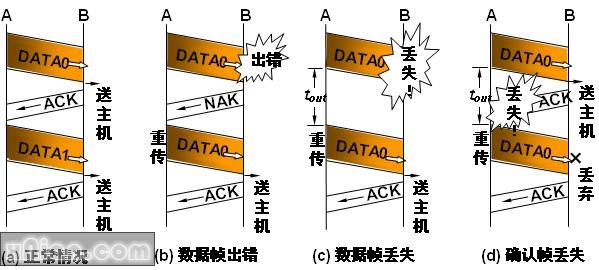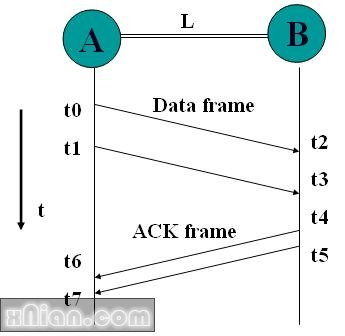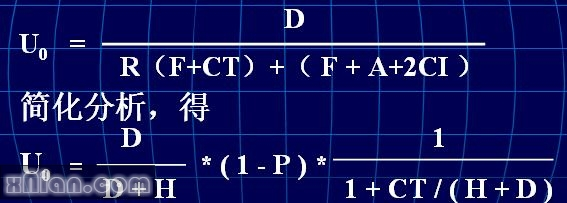# 停止等待协议t P = t2 – t0 = t3 – t1 = L / v 传播时延

t F = t1 – t0 = t3 – t2 = F / C 发送时延

t A = t5 – t4 = A / C 应答帧发送时延

t proc= t4 – t3 处理时延

L 节点A 与 B 之间的距离

v 信号传播速率 （3× 108 m / s）

F 数据帧长度 = H + D （帧头＋数据）

A 应答帧长度

C 数据发送速率 bits/s

U = t D / (tF + tA + 2tP + 2tproc)

t D = D/C ，t F = F/C， t A = A/C

U = D /（F＋A＋2C（ tP + tproc ））

tout > = 2tP